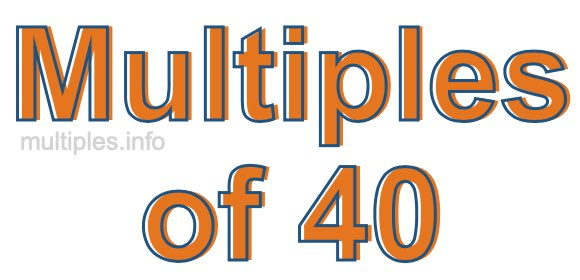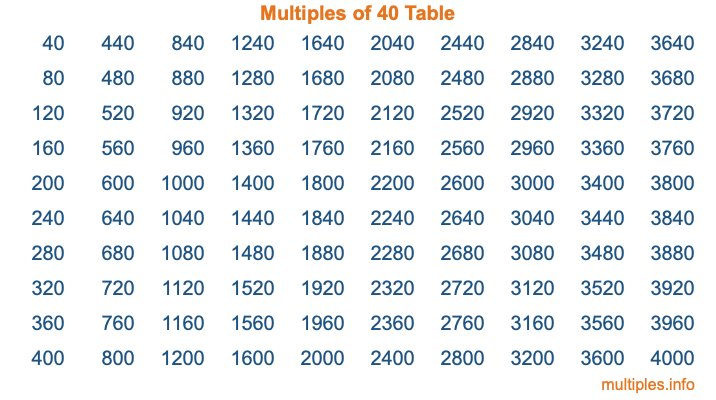Multiples of 40Welcome to the Multiples of 40 page. Here we will first teach you everything you will ever need to know about the multiples of 40, and then give you a study guide summary of everything we taught you to make sure you remember it all. Use this page to look up facts and learn information about the multiples of 40. This page will make you a multiples of forty expert!

Definition of Multiples of 40
Multiples of 40 are all the numbers that when divided by 40 equal an integer. Each of the multiples of 40 are called a multiple. A multiple of 40 is created by multiplying 40 by an integer.

Therefore, to create a list of multiples of 40, you start with 1 multiplied by 40, then 2 multiplied by 40, then 3 multiplied by 40, and so on for as long as you want. Thus, the list of the first five multiples of 40 is 40, 80, 120, 160, and 200. To see a larger list of multiples of 40, see the printable image of Multiples of 40 further down on this page. We also have a category where you can choose any nth multiple of 40.

Multiples of 40 Checker
The Multiples of 40 Checker below checks to see if any number of your choice is a multiple of 40. In other words, it checks to see if there is any number (integer) that when multiplied by 40 will equal your number. To do that, we divide your number by 40. If the the quotient is an integer, then your number is a multiple of 40.

Is  a multiple of 40?

Least Common Multiple of 40 and ...
A Least Common Multiple (LCM) is the lowest multiple that two or more numbers have in common. This is also called the smallest common multiple or lowest common multiple and is useful to know when you are adding our subtracting fractions. Enter one or more numbers below (40 is already entered) to find the LCM.

Check out our LCM Calculator if you need more details about the Least Common Multiple or if you need the LCM for different numbers for adding and subtraction fractions.

nth Multiple of 40
As we stated above, 40 is the first multiple of 40, 80 is the second multiple of 40, 120 is the third multiple of 40, and so on. Enter a number below to find the nth multiple of 40.

th multiple of 40

Multiples of 40 vs Factors of 40
40 is a multiple of 40 and a factor of 40, but that is where the similarities end. All postive multiples of 40 are 40 or greater than 40. All positive factors of 40 are 40 or less than 40.

Below is the beginning list of multiples of 40 and the factors of 40 so you can compare:

Multiples of 40: 40, 80, 120, 160, 200, etc.

Factors of 40: 1, 2, 4, 5, 8, 10, 20, 40

As you can see, the multiples of 40 are all the numbers that you can divide by 40 to get a whole number. The factors of 40, on the other hand, are all the whole numbers that you can multiply by another whole number to get 40.

It's also interesting to note that if a number (x) is a factor of 40, then 40 will also be a multiple of that number (x).

Multiples of 40 vs Divisors of 40
The divisors of 40 are all the integers that 40 can be divided by evenly. Below is a list of the divisors of 40.

Divisors of 40: 1, 2, 4, 5, 8, 10, 20, 40

The interesting thing to note here is that if you take any multiple of 40 and divide it by a divisor of 40, you will see that the quotient is an integer.

Multiples of 40 Table
Below is an image of the first 100 multiples of 40 in a table. The table is in chronological order, column by column. The first column has the first ten multiples of 40, the second column has the next ten multiples of 40, and so on.The Multiples of 40 Table is also referred to as the 40 Times Table or Times Table of 40. You are welcome to print out our table for your studies.

Negative Multiples of 40
Although not often discussed or needed in math, it is worth mentioning that you can make a list of negative multiples of 40 by multiplying 40 by -1, then by -2, then by -3, and so on, to get the following list of negative multiples of 40:

-40, -80, -120, -160, -200, etc.

Multiples of 40 Summary
Below is a summary of important Multiples of 40 facts that we have discussed on this page. To retain the knowledge on this page, we recommend that you read through the summary and explain to yourself or a study partner why they hold true.

There are an infinite number of multiples of 40.

A multiple of 40 divided by 40 will equal a whole number.

40 divided by a factor of 40 equals a divisor of 40.

The nth multiple of 40 is n times 40.

The largest factor of 40 is equal to the first positive multiple of 40.

40 is a multiple of every factor of 40.

40 is a multiple of 40.

A multiple of 40 divided by a divisor of 40 equals an integer.

40 divided by a divisor of 40 equals a factor of 40.

Any integer times 40 will equal a multiple of 40.

Multiples of a Number
Here you can get the multiples of another number, all with the same attention to detail as we did for multiples of 40 on this page.

Multiples of
Multiples of 41
Did you find our page about multiples of forty educational? Do you want more knowledge? Check out the multiples of the next number on our list!